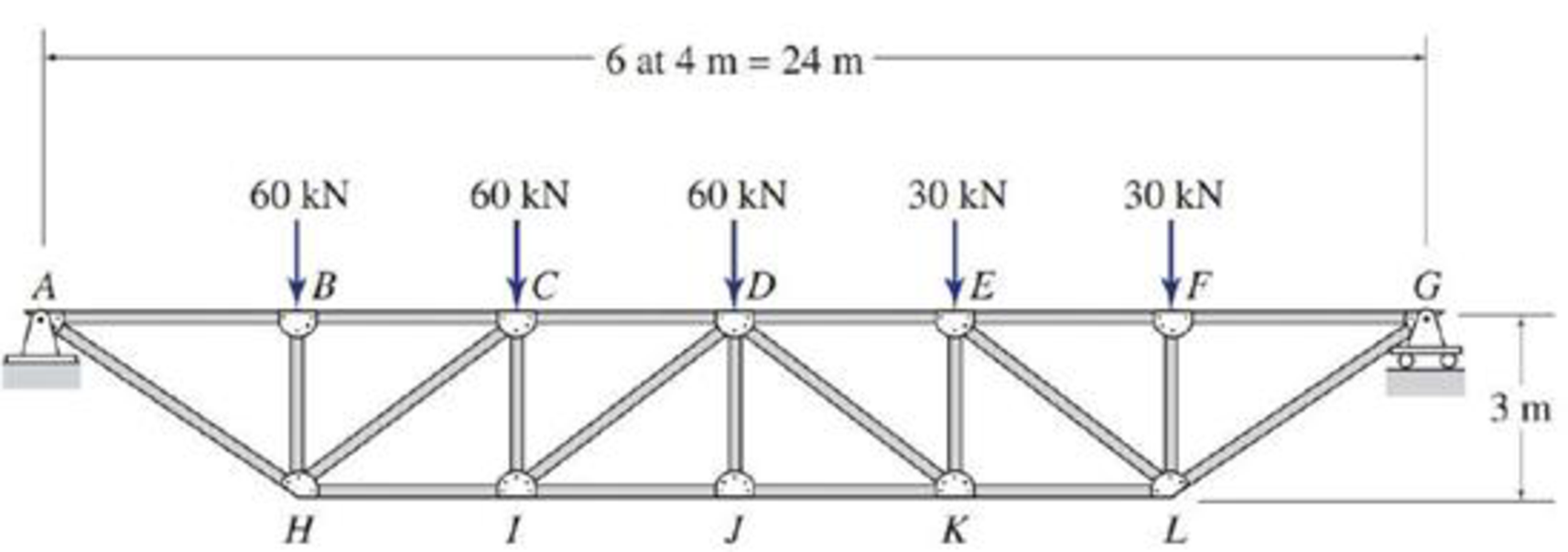# 4.6 through 4.28 Determine the force in each member of the truss shown by the method of joints. FIG. P4.14

#### Solutions

Chapter
Section
Chapter 4, Problem 14P
Textbook Problem
191 views

## 4.6 through 4.28 Determine the force in each member of the truss shown by the method of joints.FIG. P4.14

To determine

Find the forces in the members of the truss by the method of joints.

### Explanation of Solution

Given information:

Apply the sign conventions for calculating reactions, forces and moments using the three equations of equilibrium as shown below.

• For summation of forces along x-direction is equal to zero (Fx=0), consider the forces acting towards right side as positive (+) and the forces acting towards left side as negative ().
• For summation of forces along y-direction is equal to zero (Fy=0), consider the upward force as positive (+) and the downward force as negative ().
• For summation of moment about a point is equal to zero (Matapoint=0), consider the clockwise moment as negative and the counter clockwise moment as positive.

Method of joints:

The negative value of force in any member indicates compression (C) and the positive value of force in any member indicates Tension (T).

Calculation:

Consider the forces in the members AB, BC, CD, DE, EF, FG, AH, HI, IJ, JK, KL, LG, BH, CI, DJ, EK, FL, CH, DI, DK, and EL are FAB,FBC,FCD,FDE,FEF,FFG,FAH,FHI,FIJ,FJK,FKL,FLG,FBH,FCI,FDJ,FEK,FFL,FCH,FDI,FDK,FEL

Show the free body diagram of the truss as shown in Figure 1.

Refer Figure 1.

Consider the horizontal and vertical reactions at A are Ax and Ay.

Consider the vertical reaction at G is Gy.

Calculate the value of the angle θ as follows:

tanθ=34θ=tan1(34)θ=36.86°

Take the sum of the forces in the vertical direction as zero.

Fy=0Ay+Gy=60+60+60+30+30Ay+Gy=240        (1)

Take the sum of the forces in the horizontal direction as zero.

Fx=0Ax=0

Take the sum of the moments at A is zero. Then,

MA=0(60×4)(60×8)(60×12)(30×16)(30×20)+Gy×24=0240480720480600+24Gy=0Gy=252024Gy=105kN

Substitute 105kN for Gy in Equation (1).

Ay+105=240Ay=135kN

Show the joint A as shown in Figure 2.

Refer Figure 2.

Find the forces in the member AH and AB as follows:

For Equilibrium of forces,

Fy=0AyFAHsin(36.86°)=01350.6FAH=0FAH=1350.6FAH=225kN(T)

Fx=0FAHcos(36.86°)+FAB=0FAB=FAHcos(36.86°)FAB=225cos(36.86°)FAB=180kN(C)

Show the joint B as shown in Figure 3.

Refer Figure 3.

Find the forces in the member BH and BC as follows:

For Equilibrium of forces,

Fy=0FBH60=0FBH=60kNFBH=60kN(C)

Fx=0FBCFAB=0FBC=FABFBC=180kNFBC=180kN(C)

Show the joint H as shown in Figure 4.

Refer Figure 4.

Find the forces in the member HI and HC as follows:

For Equilibrium of forces,

Fy=0FHCsin(36.86°)+FHAsin(36.86°)+FHB=0FHCsin(36.86°)+225sin(36.86°)60=00.6FHC=75FHC=125kN(C)

Fx=0FHCcos(36.86°)FHAcos(36.86°)+FHI=0125cos(36.86°)225cos(36.86°)+FHI=0280+FHI=0FHI=280kN(T)

Show the joint C as shown in Figure 5.

Refer Figure 5.

Find the forces in the member CD and CI as follows:

For Equilibrium of forces,

Fy=0FCHsin(36.86°)FCI60=0125sin(36.86°)FCI60=0FCI+15=0FCI=15kN(T)

Fx=0FCB+FCDFCHcos(36.86°)=0(180)+FCD(125)cos(36.86°)=0280+FCD=0FCD=280kNFCD=280kN(C)

Show the joint I as shown in Figure 6.

Refer Figure 6.

Find the forces in the member ID and IJ as follows:

For Equilibrium of forces,

Fy=0FIDsin(36

### Still sussing out bartleby?

Check out a sample textbook solution.

See a sample solution

#### The Solution to Your Study Problems

Bartleby provides explanations to thousands of textbook problems written by our experts, many with advanced degrees!

Get Started

Find more solutions based on key concepts
Determine the natural frequency of the system given in Example 8.1 if its mass is doubled.

Engineering Fundamentals: An Introduction to Engineering (MindTap Course List)

Repeat the previous problem using sx= 12 MPa.

Mechanics of Materials (MindTap Course List)

What is the purpose of decision tables? How do you create them?

Systems Analysis and Design (Shelly Cashman Series) (MindTap Course List)

What are the steps in the SDLC?

Enhanced Discovering Computers 2017 (Shelly Cashman Series) (MindTap Course List)

What is the difference between a view and a materialized view?

Database Systems: Design, Implementation, & Management

Determine the magnitude of the pin reaction at B. Neglect the weights of the members.

International Edition---engineering Mechanics: Statics, 4th Edition

If your motherboard supports ECC DDR3 memory, can you substitute non-ECC DDR3 memory?

A+ Guide to Hardware (Standalone Book) (MindTap Course List)